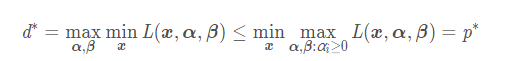## 什么是拉格朗日乘子法和KKT条件

(i) 无约束优化问题，可以写为:

​ min f(x);

(ii) 有等式约束的优化问题，可以写为:

​ min f(x),

​ s.t. hi(x) = 0; i =1, ..., n

(iii) 有不等式约束的优化问题，可以写为：

​ min f(x),

​ s.t. gi(x) <= 0; i =1, ..., n

​ hj(x) = 0; j =1, ..., m

• L(a, b, x)对x求导为零；
• h(x) =0;
• a*g(x) = 0;

## 为什么拉格朗日乘子法 和KKT条件能够得到最优值？

• f ′ ( x ) = λ g ′ ( x )
• f ′ ( y ) = λ g ′ ( y ) g ( x , y ) − c = 0
• g(x,y)−c=0

• f ′ ( x ) + λ g ′ ( x ) = 0
• f ′ ( y ) + λ g ′ ( y ) = 0
• s . t . λ ≥ 0

∇ x L ( x ∗ , α ∗ , β ∗ ) = 0 ( a )

∇ α L ( x ∗ , α ∗ , β ∗ ) = 0 ( b )

∇ β L ( x ∗ , α ∗ , β ∗ ) = 0 ( c )

α i ∗ c i ( x ∗ ) = 0 , i = 1 , 2 , ⋯ k ( d )

c i ( x ∗ ) ≤ 0 , i = 1 , 2 , ⋯ k ( e )

α i ∗ ≥ 0 , i = 1 , 2 , ⋯k ( f )

h j ( x ∗ ) = 0 , j = 1 , 2 , ⋯ l ( g )

• 条件(a)为原函数在切点的法向量被约束曲线在该点的法向量线性表出
• （b）(c） 表示最优解落在约束条件所在的曲线上
• （e）为约束条件的表达形式，都是小于等于的形式
• （f）使得原函数的法向量与约束曲线的法向量在切点处异号
• （g）为等式约束的形式

α i ∗ c i ( x ∗ ) = 0 , i = 1 , 2 , ⋯ k ( d )

## 拉格朗日对偶性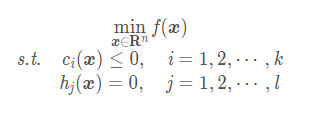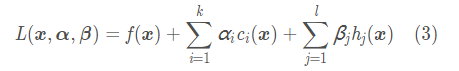• f ( x )是可微的
• α i , β j 成为拉格朗日乘子且α i ≥ 0
• c i ( x ) ≤ 0
• h i = 0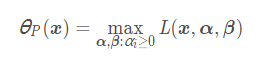• 满足原始问题的约束，这种情况下c i ( x ) ≤ 0 ,h j ( x ) = 0 ,并且α i ≥ 0 ，那我们只要让c i 的拉格朗日系数α为0，就能得到最大的结果f*(x)

• 不满足原始问题的约束，这种情况下要么 c i ( x ) > 0 或者h j ( x ) ≠ 0,那我们就能通过改变α β 使得 θ**P*(x)取到正无穷。

所以我们就有如下的式子：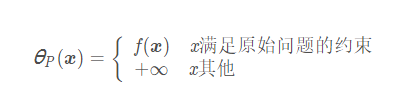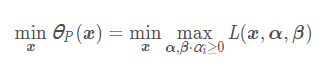p∗=xmin θ**P(x)

## 对偶问题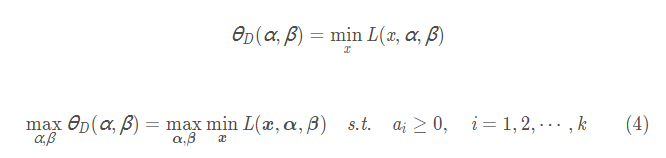d∗=α,βmax θD*(α,*β)

### 原始问题与对偶问题的关系